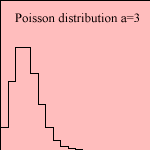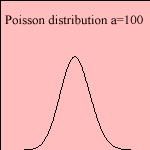# Poisson distribution

## discrete curve

last updated: 2003-08-01The Poisson distribution (Siméon Denis Poisson, 1781-1840) can be obtained from the binomial distribution: let the number of events N go to infinity, where the most probable number of successes remains constant 1).
The function is used to be normalized with a factor e-a.
It is not symmetric, as the Gaussian distribution.

We can use the Poisson distribution in the case of a discrete probability process, with a constant frequency. Examples can be found in nuclear physics while counting elementary particles.

notes

1) product of n and p, denoted as: a.